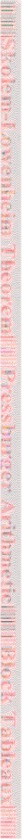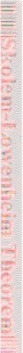back to CS486
Course HomepageSUMMARY CS 486: Applied Logic Spring 2003 Major Topics Propositional Calculi (Classical and Intuitionistic) - Smullyan book Analytic tableau Completeness and decidability Gentzen Systems/Sequents/Refinement Logics Formal proof of decidability Proof expressions Second-order propositional logic, system F Predicate Calculi (Classical and Intuitionistic) - Smullyan book Specification Language Completeness and Compactness Theorems Fundamental Theorem Church's Theorem Formal Number Theory (Peano Arithmetic (PA) and Heyting Arithmetic (HA)): Suppes book Set Theory (ZF and IZF): Suppes book Typed and Higher Order Logics (Type Theory and Class Theory): Lecture Notes and Nuprl web page Programming Logics: Lecture Notes Gödel's Theorem : Handout Special topics as possible Connection to Computer Science The driving idea is to use CS background as a springboard to get deep into parts of logic by recasting them in CS terms. This is a CS course that shows how logic is an integral part of CS (AI, systems, program verification, programming languages), and CS recasts large parts of logic in its own terms -- computabilitiy, specification, verification, model checking, intelligent systems, automated reasoning. The details will come out as we "rephrase Smullyan" and recast it. In a more ambitious course, we would recast all of Booles & Jeffrey or all of Stewart & Tall. Background Logic is concerned with propositions and proofs, just as number theory is concerned with natural numbers and operations on numbers. A proposition is an abstract mathematical object corresponding to a declarative sentence. We speak of the sense of a proposition and of its truth value. The thought expressed by a proposition is its sense, and whether the proposition is true is expressed by its truth value. Logic deals with the truth value of propositions independently of subject matter. The raw data of logic are sentences of natural languages or formulas of artificial languages, but these are representations of the abstract notion of a proposition. The truth of a proposition depends on its meaning or its sense. Access to truth is via evidence, particularly in the form of proof. Many rules of proof were discovered by the Greeks, and Aristotle organized them into a logic. The notion of proof is used also as the basis for meaning; to understand a proposition is to know how to prove it -- although one may not know whether a proof can be found. These are the basic concepts of logic. One can see that they are fundamental. This course presents precise versions of these concepts and the relationships among them. At the turn of the 20th century, Bertrand Russell showed that there is no single class of all propositions, so logic is concerned with subclasses of them organized into restricted logics. Gottlob Frege introduced the idea of using functions in the sense of mathematics to analyze the structure of propositions into operators applied to propositional functions. This leads to the study of a class of logics called functional or predicate calculi. According to Russell the domains over which a propositional function are meaningful are called types. He introduced a hierarchy of types which are used to classify functional calculi into first-order, second-order and so forth. First-order logic can express many theories of mathematics including Peano arithmetic and Zermelo-Fraenkel (ZF) set theory. Because most of mathematics can be written in ZF set theory, hence most of science can be too, first-order logic has become the focus of much research and is the logic about which the most is known; thus it is the most studied in logic courses. A distinct sublogic is the logic of pure propositional functions called the propositional calculus or Boolean algebra after George Boole who discovered it. The concept of a formal system was introduced by David Hilbert to study logics as mathematical objects. A formal system is essentially a system that can be processed by a computer. Computers can check whether a string of symbols represents a sentence and whether an expression is a legal proof? One of the enduring topics of investigation in modern logic is what operations computers can perform on formulas and proofs. For example, can a computer decide whether there is a proof of a formula and find one if there is? Can a computer list all the theorems of a logic? Kurt Gödel, Alonzo Church, and Alfred Tarksi made fundamental discoveries about formal logics. Gödel showed that even arithmetic truth cannot be completely formalized, and Tarksi showed that truth of a logic cannot in general be defined inside that logic. Church showed that computers cannot tell whether a formula of first-order logic is provable. These results will be stated precisely in the course, and Gödel's theorems will be studied. L. E .J. Brouwer, Thoralf Skolem and others discovered that many propositions in mathematics have computational meaning. Arend Heyting showed that these propositions form the core of first-order logic and arithmetic, and that Peano arithmetic (PA) can be factored into Heyting arithmetic (HA) and Aristotle's axiom of excluded middle. N.G. deBruijn generalized this computational meaning by defining a proposition as the type of its proofs. This computational meaning can be expressed in programming languages, and Alan Turing claimed as a "thesis" that the computational meaning of mathematics is given precisely by Turing machines (or modern digital computers). Alonzo Church proposed an equivalent thesis that effective computability was captured by his lambda calculus (modern high-level programming languages) which can be "compiled" to Turing machines as computer scientist have shown. Computer scientists have also shown how to implement this computational core of logics as a programming language and reasoning system combined into one language. This was first done for a theory based on Per Martin-Lof's type theories. Such mathematical systems form a rich class of programming logics which have proven useful in computer science. Conversely, advances in computer science have created a new field of mathematics called Formalized Mathematics. This course will demonstrate these connections between computer science and logic. Goals At the end of the course, students should be able to discuss at length the relationship between logic and computer science stressing connections to functional programming (illustrated for the ML language). They should know a variety of ways to formally specify mathematical and computational problems as well as a variety of techniques for formally proving these specifications. Additionally, students should know the statement and significance of several major theorems in logic including Gödel's Completeness and Incompleteness Theorems, Church's Theorem and Tarski's Theorem on truth.
back to CS486
Course Homepage

 Webms: Juanita Heyerman, Department of Computer Science, Cornell University, Ithaca, NY. Mail to: juanita@cs.cornell.edu